A new idea to obtain clean fusion energy

11.14 Confinement of positive ions and electrons with a static electric and magnetic fieldExperiments with the simulation program: exp. 11.14..11.17

Back to main page

 Experiment 11.14a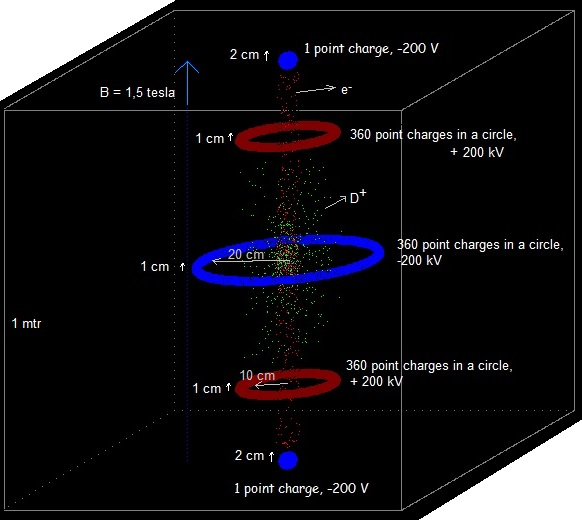ve:=6E6; hydrogen[i].vx:=0 + (-0.5 + random)*ve;  {= deuterium ion} hydrogen[i].vy:=0+( - 0.5 + random)*ve;  {< 3E6 m/s} hydrogen[i].vz:=0 +( - 0.5 + random)*ve; hydrogen[i].x:=0.5+ ( - 0.5 + random) /20; {so they start not in exactly the same point} hydrogen[i].y:=0.5+ ( - 0.5 + random) /20; hydrogen[i].z:=0.5 + ( - 0.5 + random) /20; hydrogen[i].m:=mp; mp=2*1.6726E-27; electron[i].vx:=0 + (-0.5 + random)*ve;  {< 3E6 m/s} electron[i].vy:=0 + (-0.5 + random)*ve;; electron[i].vz:=0 + (-0.5 + random)*ve; electron[i].x:=0.5+ ( - 0.5 + random) /20; electron[i].y:=0.5+ ( - 0.5 + random) /20; electron[i].z:=0.5+ ( - 0.5 + random) /20; B-field = 1,5 tesla ( vertical) Dimensions of the rings and spheres as indicated in the above image. Program running about 48 hrs, with dt= 1E-11 and dt=1E-10 s. Total energy   8,9E-12 J < Etotal < 9,2 E-12 J (probably an error in the program, will check this out) Checked it out and the total energy is not right calculated. (because I changed the fixed charges) Both the positive D+ ions and the electrons  stay (more or less) confined in the simulation space! Programmed the checking of the maximum distance of the electrons and the D+ ions from the vertical central axis. During the simulation time this distance did not change significant (so it seems to be that they did not spread out sidewards):. Screenshot 8.74E-6  s Experiment 11.15a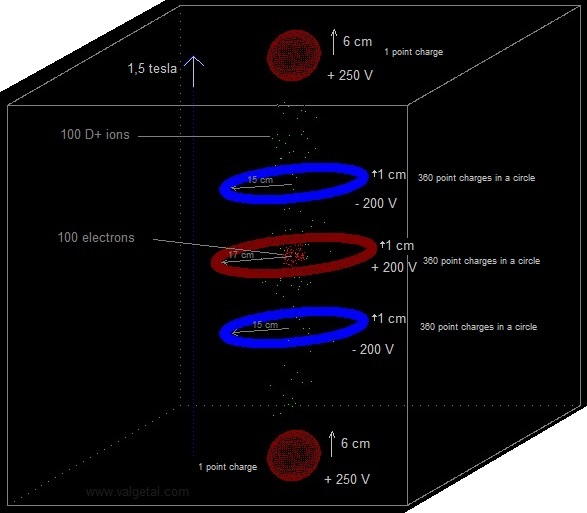ve:=6E6; hydrogen[i].vx:=0 + (-0.5 + random)*ve;  {= deuterium ion} hydrogen[i].vy:=0+( - 0.5 + random)*ve;  {< 3E6 m/s} hydrogen[i].vz:=0 +( - 0.5 + random)*ve; hydrogen[i].x:=0.5+ ( - 0.5 + random) /20; {so they start not in exactly the same point} hydrogen[i].y:=0.5+ ( - 0.5 + random) /20; hydrogen[i].z:=0.5 + ( - 0.5 + random) /20; hydrogen[i].m:=mp; mp=2*1.6726E-27; electron[i].vx:=0 + (-0.5 + random)*ve;  {< 3E6 m/s} electron[i].vy:=0 + (-0.5 + random)*ve;; electron[i].vz:=0 + (-0.5 + random)*ve; electron[i].x:=0.5+ ( - 0.5 + random) /20; electron[i].y:=0.5+ ( - 0.5 + random) /20; electron[i].z:=0.5+ ( - 0.5 + random) /20; B-field = 1,5 tesla ( vertical) Dimensions of the rings and spheres as indicated in the above image. Exp 11.15 screenshot 3.45E-6 s With slightly different voltages and dimensions: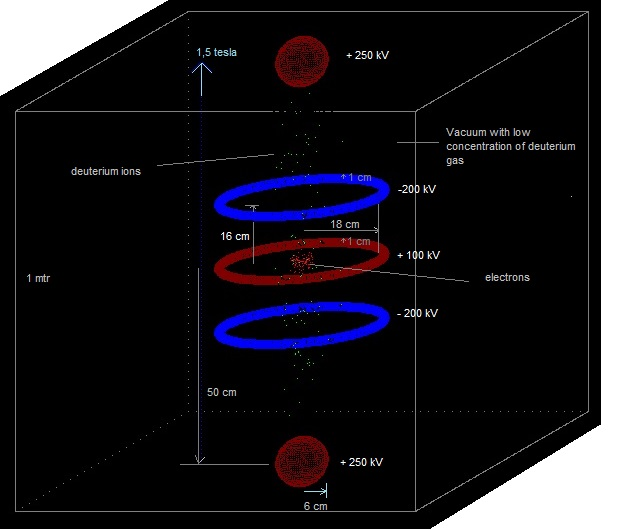Experiment 11.16 B = 1 tesla Voltages = +/- 50 kV No initial speed of the particles: hydrogen[i].vx:=0; {deuterium!} hydrogen[i].vy:=0; hydrogen[i].vz:=0; hydrogen[i].x:=0.5+ ( - 0.5 + random) /20; {so they start not in exactly the same point} hydrogen[i].y:=0.5+ ( - 0.5 + random) /20; hydrogen[i].z:=0.5 + ( - 0.5 + random) /20; hydrogen[i].m:=mp; mp=2*1.6726E-27; electron[i].vx:=0; electron[i].vy:=0; electron[i].vz:=0; electron[i].x:=0.5; electron[i].y:=0.5; electron[i].z:=0.5; 100 D+ and 100 e- generated, they all stay confined. Exp 11.16 screenshot Because the initial speed of the particles is zero, the electric field can be less (less voltage). Experiment 11.17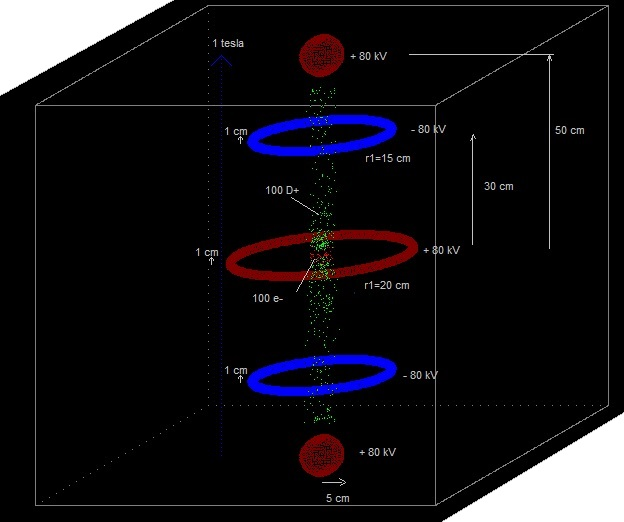B = 1 tesla Voltages = +/- 80 kV (inverted!) No initial speed of the particles: hydrogen[i].vx:=0; {deuterium!} hydrogen[i].vy:=0; hydrogen[i].vz:=0; hydrogen[i].x:=0.5+ ( - 0.5 + random) /20; {so they start not in exactly the same point} hydrogen[i].y:=0.5+ ( - 0.5 + random) /20; hydrogen[i].z:=0.5 + ( - 0.5 + random) /20; hydrogen[i].m:=mp; mp=2*1.6726E-27; electron[i].vx:=0; electron[i].vy:=0; electron[i].vz:=0; electron[i].x:=0.5; electron[i].y:=0.5; electron[i].z:=0.5; 100 D+ and 100 e- generated, they all stay confined. Exp 11.17 screenshot Because the initial speed of the particles is zero, the electric field can be less (less voltage). The electrons get very hign speed in the vertical directions (more than speed of light). We should adapt the formulas in the program for relativistic speeds. Experiment 11.18ve:=6E6; hydrogen[i].vx:=0 + (-0.5 + random)*ve;  {= deuterium ion} hydrogen[i].vy:=0+( - 0.5 + random)*ve;  {< 3E6 m/s} hydrogen[i].vz:=0 +( - 0.5 + random)*ve; hydrogen[i].x:=0.5+ ( - 0.5 + random) /20; {so they start not in exactly the same point} hydrogen[i].y:=0.5+ ( - 0.5 + random) /20; hydrogen[i].z:=0.5 + ( - 0.5 + random) /20; hydrogen[i].m:=mp; mp=2*1.6726E-27; intial speed  <= 3E6 m/s electron[i].vx:=0 + (-0.5 + random)*ve;  {< 3E6 m/s} electron[i].vy:=0 + (-0.5 + random)*ve;; electron[i].vz:=0 + (-0.5 + random)*ve; electron[i].x:=0.5+ ( - 0.5 + random) /20; electron[i].y:=0.5+ ( - 0.5 + random) /20; electron[i].z:=0.5+ ( - 0.5 + random) /20; B-field = 1 tesla ( vertical) Dimensions of the rings and spheres, see screenshot. Voltages: +/- 100 kV Generated 5729 electrons and 20 D+. The program did not proceed anylonger because of too many particles.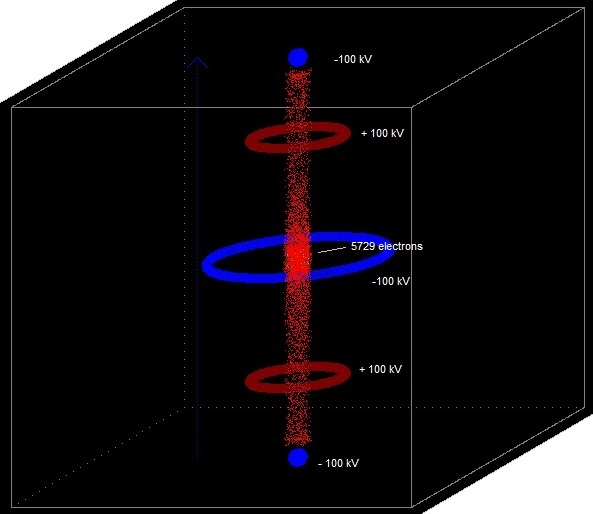5729 electrons x 1,16E-19 C = 6,6E-16 C. If this charge would be on a sphere with a radius of 5 cm, the voltage of it would be: V = 9E9. 6,6E-16 / 0,05 = 1,2E-4 V. This voltage is negligible compared with the voltages of the rings and the up/down spheres of the fusor device. What can be seen is that the electrons seem to concentrate in the centrum (understandable, because they slow down there).  In a real device they could perhaps form a virtual cathode and atract the D+ ions also more towards the centre. Experiment 11.19 ve:=3E6; hydrogen[i].vx:=0 + (-0.5 + random)*ve;  {= deuterium ion} hydrogen[i].vy:=0+( - 0.5 + random)*ve;  {< 3E6 m/s} hydrogen[i].vz:=0 +( - 0.5 + random)*ve; hydrogen[i].x:=0.5+ ( - 0.5 + random) /20; {so they start not in exactly the same point} hydrogen[i].y:=0.5+ ( - 0.5 + random) /20; hydrogen[i].z:=0.5 + ( - 0.5 + random) /20; hydrogen[i].m:=mp; mp=2*1.6726E-27; initial speed < 1,5E6 m/s electron[i].vx:=0 + (-0.5 + random)*ve;  {< 3E6 m/s} electron[i].vy:=0 + (-0.5 + random)*ve;; electron[i].vz:=0 + (-0.5 + random)*ve; electron[i].x:=0.5+ ( - 0.5 + random) /20; electron[i].y:=0.5+ ( - 0.5 + random) /20; electron[i].z:=0.5+ ( - 0.5 + random) /20; B-field = 1 tesla ( vertical) Dimensions of the rings and spheres, see screenshot. Voltages: +/- 80 kV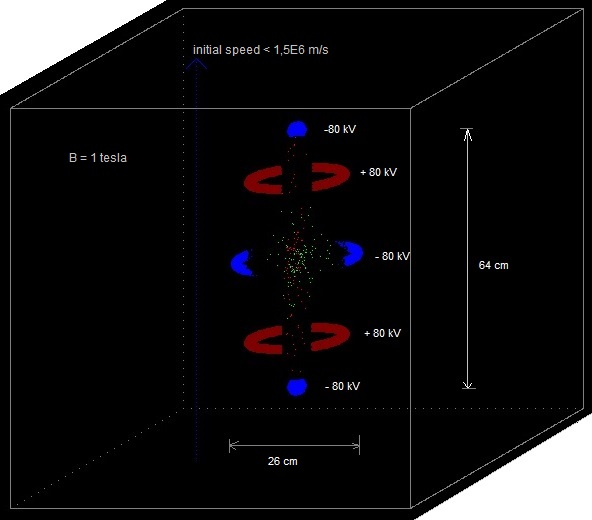If the random initial speed is taken less,  the voltages and the dimensions can decrease. Experiment 11.20 ve=1E6 m/s B= 0.6 tesla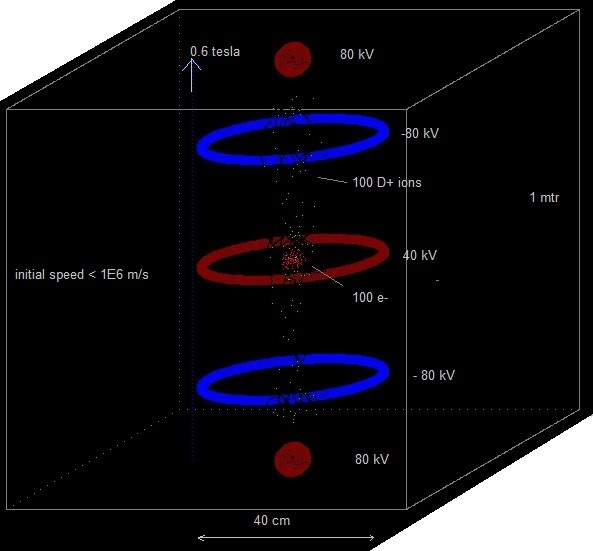Experiment 11.21 Particles generated in the centre region with initial random speed in all directions < 3,3E6 m/s B= 1,6 tesla Elapsed time = 4,72 E-5 s (simulation run about 24 hrs)   The total energy of the particles and the maximum radii of the electrons and the D+ ions stayed constant  during more or less the last part of the experiment. The total energy has to stay constant (bremsstrahlung and relativistic effects are not taken into account), so the simulation (leapfrog integration) seems to work correctly. Because the maximum radii did not increase, the particles seem not to escape, which is also good.

20 February 2014 ..     by  Rinze Joustra        www.valgetal.com